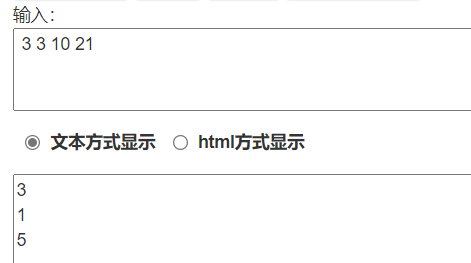2021-10-04 20:25

51nod找数字 V2 （小学生发布请多多关照，代码写了五十多行但超时）

• 写回答
• 好问题 提建议
• 追加酬金
• 关注问题
• 收藏
• 邀请回答

2条回答默认 最新

•已采纳

这么写，如果有帮助请点一下我回答右上方的采纳，谢谢！以后有什么问题可以互相交流。#include<bits/stdc++.h>
#include <cmath>
using namespace std;
int n,sheng,k,m,f,weinum=0,i=1,mua;//i是第几位，
int mink;
unsigned long long wei(int k) {
int num=9*k*pow(10,k-1);
return num;
}
void qiumink(int n) {
int num=1;
for(int i=2; i<=n; i++)
num=num*10;
num--;
mink=num;
}
int weishu(int mink,int i) {
if(i==0){
return mink%10;
}
int a=mink,b=0;
while(a!=0) {
a=a/10;
b++;
}
if(b==1)
return mink;

for(int n=1; n<=abs(b-i); n++) {
mink=mink/10;
}
return mink%10;
}
int main() {
cin>>f;
for(int o=1; o<=f; o++) {
cin>>n;
while(n>=weinum) {
weinum=wei(i)+weinum;
i++;
}
i--;
weinum=weinum-wei(i);
sheng=n-weinum;
k=ceil((sheng*1.0)/i);
qiumink(i);
mink+=k;
mua=sheng%i;
cout<<weishu(mink,mua)<<endl;
weinum=0,i=1;
}
return 0;
}

评论
解决 无用
打赏 举报